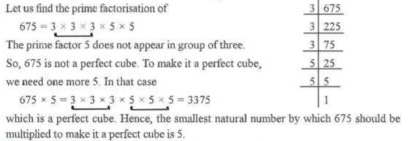# Exercise 6.3 class 8 solution

#### Exercise 6.3 class 8 solution-Introduction

Welcome to our website, your one-stop destination for free mathematics solutions for Class 8! students can easily download Exercise 6.3 class 8 solution pdf here as well as other chapter solution pdfs.

We understand that the journey through mathematics can be both exciting and challenging for students at this crucial stage of their academic growth. That’s why we’re here to offer comprehensive solutions that simplify complex concepts, provide step-by-step guidance, and build confidence in tackling mathematical problems.

Our mission is to empower Class 8 students with the knowledge and resources they need to excel in mathematics. Whether you’re seeking assistance with algebra, geometry, or any other math topic, our carefully crafted solutions are designed to make learning engaging and accessible.

#### Exercise 6.3 class 8 solution- Find smallest multiple

In last section we have observed that every number is not a perfect cube. If a number is not a perfect cube, we can find the smallest natural number by which the given number must be multiplied, so that the product is a perfect cube. We can also find the smallest number to divide the given number, so that quotient is a perfect cube.

#### Exercise 6.3 class 8 solution -examples of the exercise

Example 6.3.1. Is 243 a perfect cube? If not,find the smallest number by which 243 must be multiplied so that the product is a perfect cube. Also find the number.

Solve.

The prime factorization of 243 is 3x3x3x3 × 3 = 3^3 × 3^2

The prime factor 3 does not appear in a group of three. So 243 is not a perfect cube. To make it perfect cube, we need one more 3, so to make 243 a perfect cube. we have to multiply it by 3.

243* 3=729

3х3х3х3х3х3=729

Now, it is a perfect cube number. Hence, the smallest number to be multiplied is 3 and 729 is the number which is perfect cube.

Example 6.3.2 Is 675 a perfect cube? If not, find the smallest natural number by which 675 must be multiplied so that the product is a perfect cube.Example 6.3.3 Is 31944 a perfect cube? If not, then by which smallest natural number should 31944 be divided so that quotient is a perfect cube?

Solve.

Hence the smallest number by which 31944 should be divided to make it a perfect cube is 3.

#### Exercise 6.3 class 8 solution- exercise preview

Our Exercise 6.3 Preview is designed to give you a taste of what’s in store. You’ll find a selection of math problems and questions that challenge your analytical and problem-solving skills. It’s a glimpse into the wonderful world of math exercises that will not only sharpen your mathematical prowess but also help you build the confidence you need to excel in your Class 8 studies.

These exercises are carefully curated to cover a range of mathematical concepts, from basic to more advanced topics. Whether you’re preparing for an upcoming exam, aiming for a deeper understanding of math, or just love a good mathematical challenge, this preview is the perfect starting point.

#### Exercise 6.3 class 8 solution- solution pdf

We understand that sometimes math can be a puzzle, and that’s where we come in. Our user-friendly platform offers you the convenience of accessing Exercise 6.3 class 8 solutions in just a few clicks. No more flipping through textbooks or endless internet searches—your math solutions are right here, waiting for you .

These downloadable resources are designed to simplify complex concepts, provide clear step-by-step explanations, and boost your confidence in solving math problems. We believe that learning mathematics should be accessible and enjoyable, and we’re committed to making it so.

So, get ready to unlock the world of mathematics and explore Exercise 6.3 class 8 solutions that will help you not only in your exams but also in building a strong foundation for future mathematical adventures. Click, download, and excel in math! Your math journey is about to get a whole lot easier.

6.3

Exercise 7.6 class 8 solution

Exercise 7.8 class 8 solution

Exercise 9.2 class 8 solution

Exercise 9.5 class 8 solution

Exercise 10.2 class 8 solution

Exercise 12.1 class 8 solution

Exercise 12.3 class 8 solution

https://nautiyalqueen.blogspot.com/2022/01/heal-yourself.html

https://nautiyalqueen.blogspot.com/2022/01/beauty.html